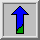# Distance Transform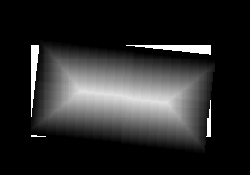Common Names: Distance transform

## Brief Description

The distance transform is an operator normally only applied to binary images. The result of the transform is a graylevel image that looks similar to the input image, except that the graylevel intensities of points inside foreground regions are changed to show the distance to the closest boundary from each point.

One way to think about the distance transform is to first imagine that foreground regions in the input binary image are made of some uniform slow burning inflammable material. Then consider simultaneously starting a fire at all points on the boundary of a foreground region and letting the fire burn its way into the interior. If we then label each point in the interior with the amount of time that the fire took to first reach that point, then we have effectively computed the distance transform of that region. Figure 1 shows a distance transform for a simple rectangular shape.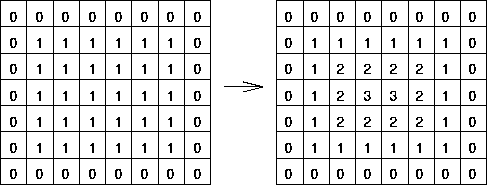Figure 1 The distance transform of a simple shape. Note that we are using the `chessboard' distance metric.There is a dual to the distance transform described above which produces the distance transform for the background region rather than the foreground region. It can be considered as a process of inverting the original image and then applying the standard transform as above.## How It Works

There are several different sorts of distance transform, depending upon which distance metric is being used to determine the distance between pixels. The example shown in Figure 1 uses the `chessboard' distance metric but both the Euclidean and `city block' metrics can be used as well.

Even once the metric has been chosen, there are many ways of computing the distance transform of a binary image.One intuitive but extremely inefficient way of doing it is to perform multiple successive erosions with a suitable structuring element until all foreground regions of the image have been eroded away. If each pixel is labeled with the number of erosions that had to be performed before it disappeared, then this is just the distance transform. The actual structuring element that should be used depends upon which distance metric has been chosen. A 3×3 square element gives the `chessboard' distance transform, a cross shaped element gives the `city block' distance transform, and a disk shaped element gives the Euclidean distance transform. Of course it is not actually possible to generate a good disk shaped element on a discrete grid on a small scale, but there are algorithms that vary the structuring element on each erosion so as to approximate a circular element.The distance transform can be calculated much more efficiently using clever algorithms in only two passes (e.g. Rosenfeld and Pfaltz 1968). This algorithm, which is based on recursive morphology, will not be described here.## Guidelines for UseThe distance transform is very closely linked to both the medial axis transform and to skeletonization. It can also be used to derive various other symmetries from binary shapes. As such it is usually only used as a step on the way to producing these end products (and in fact is often only produced in theory rather than in practice).

Here we illustrate the Euclidean distance transform with some examples.

The binary imagebecomeswhen a distance transform is applied (scaled by a factor of 5).

Similarly,becomes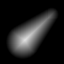(scaled by a factor of 3).

And finally,becomes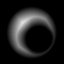(scaled by a factor of 4).The distance transform is sometimes very sensitive to small changes in the object. If, for example, we change the above rectangle towhich contains a small black region in the center of the white rectangle, then the distance transform becomes(after brightening the image by a factor of 6). This can be of advantage when we want to distinguish between similar objects like the two different rectangles above. However, it can also cause problems when trying to classify objects into classes of roughly the same shape. It also makes the distance transform very sensitive to noise. For instance, if we add some `pepper noise' to the above rectangle, as inthe distance transform yields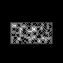(brightened by a factor of 15).

An example of applying the distance transform to a real world image is illustrated withTo obtain a binary input image, we threshold the image at a value of 100, as shown inThe scaled (factor 6) distance transform is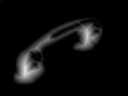Although the image gives a rough measure for the width of the object at each point, it is quite inaccurate at places where the object is incorrectly segmented from the background.

The last three examples show that it is important that the binary input image is a good representation of the object that we want to process. Simple thresholding is often not enough. It might be necessary to further process the image before applying the distance transform.

## Interactive Experimentation

You can interactively experiment with this operator by clicking here.

## Exercises

1. Try to obtain a better binary image of the telephone receiver so that the distance transform gives a better result. Consider applying some other morphological operators (e.g. closing) to the thresholded image.

2. Imagine representing the distance transform in 3-D, i.e. displaying the distance to the nearest boundary point on a third axis. What shape is the Euclidean distance transform of a circle?

3. Discuss the differences between the distance transforms using `city block', `chessboard' and Euclidean distance metrics. Under what situations are they different from each other? How do they vary on the example images above?

4. Why might you choose to use one distance metric over another?

## References

A. Jain Fundamentals of Digital Image Processing, Prentice-Hall, 1989, Chap. 9.

R. Haralick and L. Shapiro Computer and Robot Vision, Vol. 1, Addison-Wesley Publishing Company, 1992, Chap. 5.

A. Rosenfeld and J. Pfaltz Distance Functions in Digital Pictures, Pattern Recognition, Vol. 1, 1968, pp 33 - 61.

## Local Information